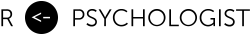# Creating a typical textbook illustration of statistical power using either ggplot or base graphics

May 26, 2013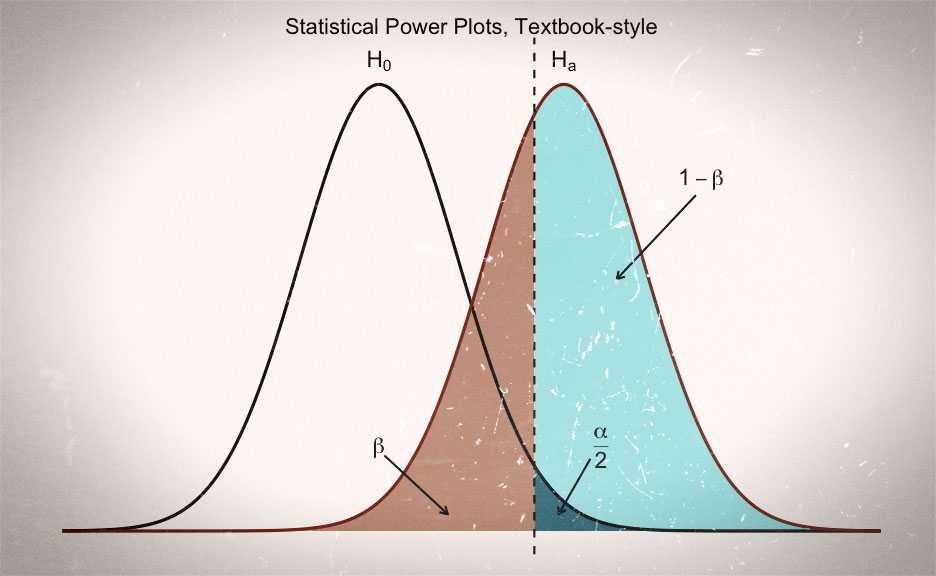A common way of illustrating the idea behind statistical power in null hypothesis significance testing, is by plotting the sampling distributions of the null hypothesis () and the alternative hypothesis (). Typically, these illustrations highlight the regions that correspond to making a type II error (), type I error () and correctly rejecting the null hypothesis (i.e. the test’s power; ).

In this post I will show how to create such “power plots” using R. Typically, I prefer to use ggplot for plotting, but tasks such as this is one of the few times were I think R’s base graphics have some merit—especially for creating black and white plots, since ggplot does not support using patterns. Thus, I will present code both for ggplot and base graphics.

Creating these plots is pretty straight forwards. You only need to be vaguely familiar with the mechanics of plotting polygons. For instance, say we want to plot a triangle with the following coordinates.

Then we just specify x and y as vectors, like this: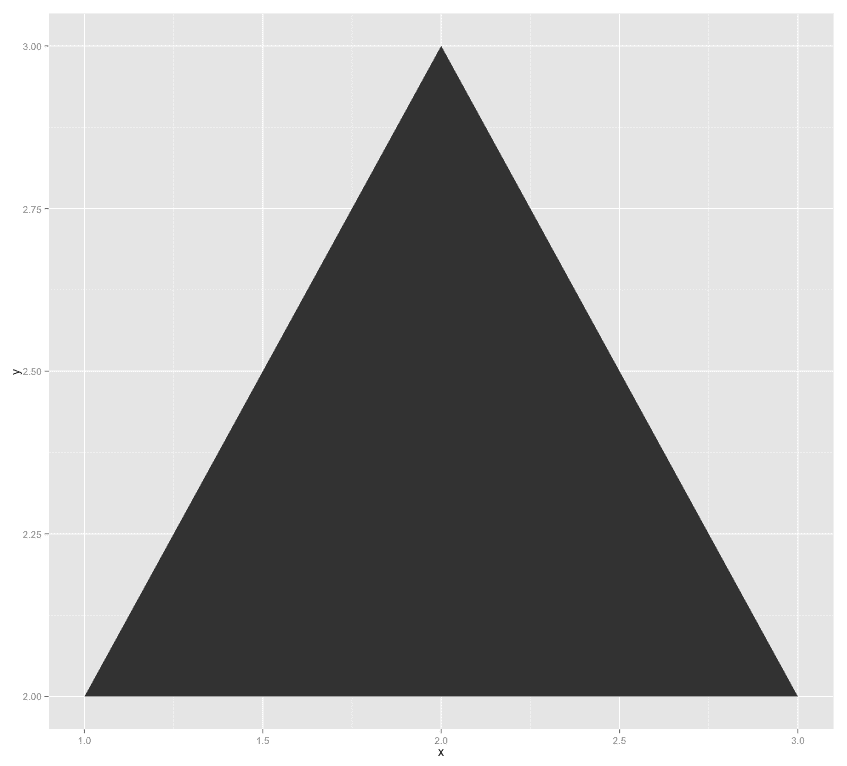So, let us begin by creating the data for the two distributions and three polygons that we will need.

Now that we have all the data that we need, let us create the first plot using ggplot. The annotation is set manually, so it will be a bit tedious to change these plots.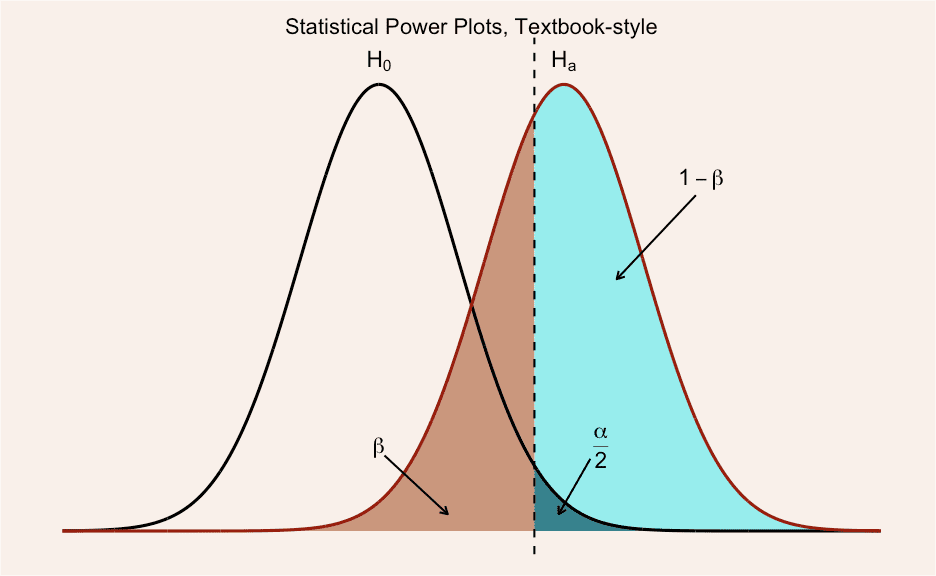Now, if we want a more “classical looking” black and white-plot, we need to use base graphics.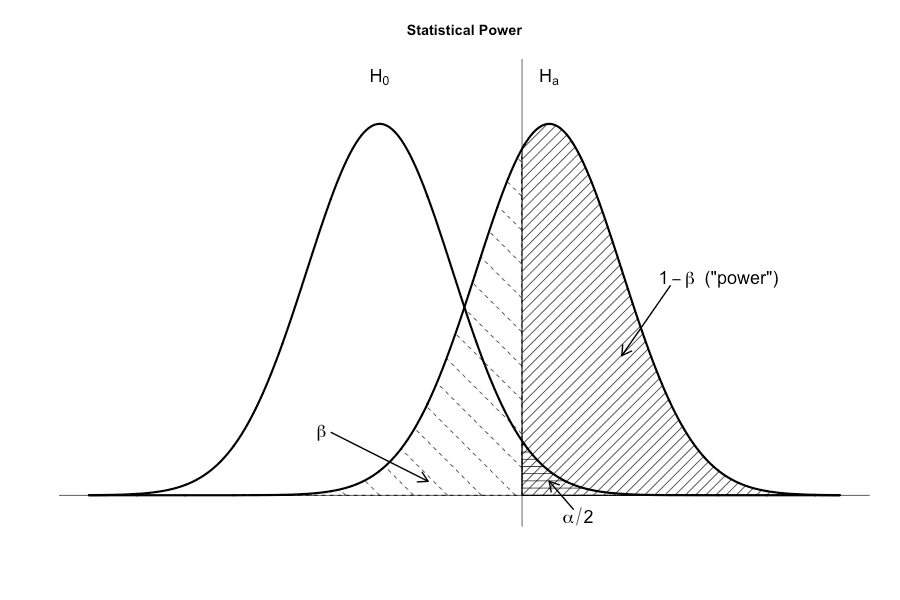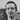Written by Kristoffer Magnusson a researcher in clinical psychology. You should follow him on Twitter and come hang out on the open science discord Git Gud Science.

Share:

Published May 26, 2013 (View on GitHub)